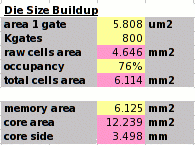This page describes how to convert the basic chip specifications into an estimate for the core size. The chip size is then the core size plus the height of the pad library plus the scribe line width. The pads and scribe values are know. What we have to estimate is the core size.

On this page we estimate the core size with no added power straps. The following pages will estimate the amount of metal to add for the power straps and its impact on the core size.

The user estimates the design to have 800K gates and memory blocks which have an area of 6.125mm². The standard cell library is the wsclib in 0.13µm with a value of lambda = 0.055µm. The cell height is 80λ (4.4µm) or 10 tracks. The area of one gate is 24×80×0.0552=5.808µm2.

The memory blocks are surrounded by metal supply rings which allow the standard cell rows to butt right up against the memories. If the memories don't have these rings, then their area should be added to their size estimate. This factor is sometimes called the block routing adder.

Even if the standard cell rows butt up to the memories, it is likely that cells cannot be placed very close because of routing congestion. This is handled by setting an occupancy for the standard cells. We fill the available core with standard cell rows (a sea of gates approach) and then say what percentage will be occupied by standard cells.

Guessing a good number for this occupancy is the real skill here. I think values between 75%-85% are normally achieved. Larger circuits will have a lower occupancy, and circuits with lots of small memory blocks will also have lower occupancy.

In this example, the designer estimates he can achieve a 76% occupancy.

This means the standard cell area in square mm is

 800,000 × 5.808 ⁄ 0.76 ⁄ 1,000,000 = 6.114mm2.

The core area is the sum of the memory and the standard cell areas

 6.125 + 6.114 = 12.239mm2.

The core side is the square root of this, or 3.5mm.

### A note on the definition of a gate

Some care must be taken with the definition of a gate when estimating the area of integrated circuits. There are three common usages.
1. A gate is a cell which is 3 routing tracks wide and can have as many as 3 connectors. The cell width in tracks is divided by 3 for its gate count.
2. A gate is the width of a basic 2−NAND cell. A cell's width is divided by the 2−NAND width to get its gate count.
3. A gate is four transistors. Each cell's transistor count is divided by 4 to get its gate count.

The convention used here is the first one above. This is the one that maps most easily from gate count to physical area. If a basic 2−NAND gate is 3 tracks wide then the first two conventions above are the same.

Many libraries have a 2−NAND gate which is 4 tracks wide, or even 5 tracks. Gate estimates for these libraries using convention 2 above will give significantly fewer gates.

Convention 3 above is more complicated to convert a gate count to area. Considering the wsclib for example, the basic 2−NAND is 4 tracks wide. The D‑flip‑flop has 24 transistors and is 18 tracks wide.

So the 2−NAND is one gate, four tracks wide. The flop is six gates, 18 tracks wide. The factor converting from gate count to physical area is not the same for each cell.